# Island Rotation Frequency

Equations (8.101) and (9.3) imply that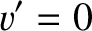(9.27)

for an isolated magnetic island chain (i.e., a chain that is not interacting with a resistive wall or an error-field). It follows from Equations (8.75), (8.76), (8.83), and (8.87) that the stream-functions for the electron, MHD, and ion fluids take the respective forms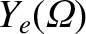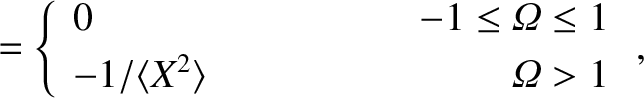(9.28)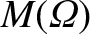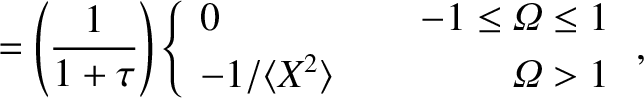(9.29)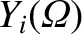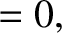(9.30)

in the vicinity of the island chain. Here,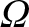is a magnetic flux-surface label that takes the value zero at the island O-points, unity on the magnetic separatrix, and is much larger that unity far from the island chain [see Equation (8.55)]. Moreover,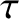is ratio of the electron and ion pressure gradients at the rational surface [see Equation (4.5)]. The function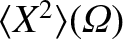is specified in Section 8.11. Finally, the poloidal components of the perpendicular velocities of the three fluids in the island rest frame are related to their stream-functions according to Equations (8.72)–(8.74).

Figure 9.3 shows typical normalized perpendicular velocity profiles of the electron, MHD, and ion fluids across the O-points and the X-points of an isolated magnetic island chain in the island rest frame, as determined from Equations (8.72)–(8.74) and (9.28)–(9.30) . Here,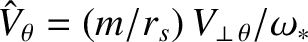, where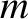is the poloidal mode number of the tearing mode, and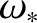is the diamagnetic frequency at the rational surface [see Equation (5.47)]. It can be seen that all three fluids co-rotate with the island chain inside the magnetic separatrix. The ion fluid velocity profile is completely unaffected by the presence of the chain. On the other hand, the electron and the MHD fluid velocity profiles are modified by the island chain. In particular, both profiles are discontinuous across the magnetic separatrix. As was explained in Section 8.9, these discontinuities are resolved in a thin layer of thickness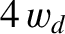on the separatrix [see Equation (8.84) and Table 8.1]. It is clear from the figure that the island chain rotates in the ion diamagnetic (i.e., negative) direction with respect to the unperturbed E-cross-B frame at the rational surface .

Equations (8.45), (8.63), (9.27), and (9.29) yield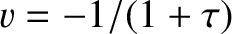, or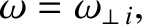(9.31)

where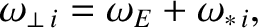(9.32)

and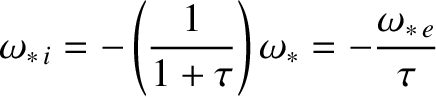(9.33)

is the ion diamagnetic frequency at the rational surface [see Equations (5.45) and (5.46)]. We conclude that, unlike a linear tearing mode, which is convected by the electron fluid at the rational surface (see Section 6.2), a nonlinear tearing mode is convected by the ion fluid . The reason for this difference is discussed in Section 1.12. Incidentally, there is clear experimental evidence that wide magnetic island chains in tokamak plasmas rotate in the ion diamagnetic direction relative to the E-cross-B frame at the rational surface [1,11].

Finally, it is reasonable to suppose that the critical island width that must be exceeded before a magnetic island chain switches from rotating in the electron direction, with respect to the unperturbed local E-cross-B frame, to rotating in the ion direction is that above which the pressure profile flattens within the separatrix .